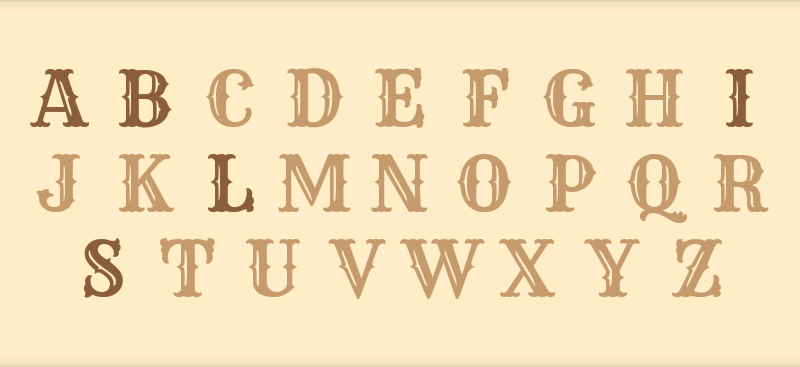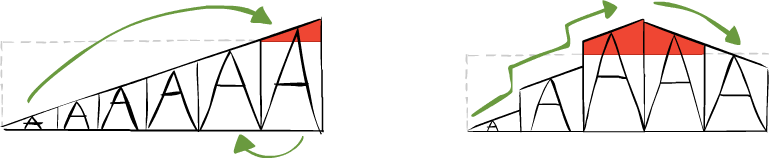# Resizing text to fit into a container on Android

A recent Android project of mine required the creation of a method for automatically resizing text so that it would fit snugly into a variable sized container. Once the optimum size had been found it could then accurately paint the text on a canvas.

Although there are solutions available for the TextView I wanted the solution to be as simple as possible, without the overhead of using a TextView. In the end what I came up with seems to work quite well, though it isn’t as deterministic as I’d hoped.

Below is an example of what the end result looks like after determining the size of each letter based on multiple containers of equal size.The solution, which was inspired by a blog post on measuring text, is to repeatedly increase the text’s size until it overlaps the boundaries of the container, then decrease its size to just before it overlapped the boundary.This was then optimized by making larger increments in font size - given the high-density of device screens nowadays - so that the larger font sizes are reached with fewer iterations. This means that font-size of 101dp can be reached in just 19 iterations, which in realtime is jolly fast.

``````private static float calculateFontSize(@NonNull Rect textBounds, @NonNull Rect textContainer, @NonNull String text) {

// Further optimize this method by passing in a reference of the Paint object
// instead of instantiating it with every call.
final Paint textPaint = new Paint();

int stage = 1;
float textSize = 0;

while(stage < 3) {
if (stage == 1) textSize += 10;
else
if (stage == 2) textSize -= 1;

textPaint.setTextSize(textSize);
textPaint.getTextBounds(text, 0, text.length(), textBounds);

textBounds.offsetTo(textContainer.left, textContainer.top);

boolean fits = textContainer.contains(textBounds);
if (stage == 1 && !fits) stage++;
else
if (stage == 2 &&  fits) stage++;
}

return textSize;
}
``````

This is what it looks like in an unoptimised example.

``````public class ExampleView extends View {

private final Paint textPaint = new Paint();

private final Rect drawableContainer = new Rect();

private final Rect boundaryOfText = new Rect();

private final String text = "Hello World";

public AlphabetBoardView(Context context, AttributeSet attrs) {
super(context, attrs);

textPaint.setTextAlign(Paint.Align.CENTER);
}

@Override
protected void onSizeChanged (int w, int h, int oldw, int oldh) {
super.onSizeChanged(w, h, oldw, oldh);

if (w > 0 && h > 0) {
drawableContainer.set(0, 0, w, h);

float fontSize = calculateFontSize(boundaryOfText, drawableContainer, text);
textPaint.setTextSize(fontSize);
}

invalidate();
}

private static float calculateFontSize(@NonNull Rect textBounds, @NonNull Rect textContainer, @NonNull String text) {

final Paint textPaint = new Paint();

int stage = 1;
float textSize = 0;

while(stage < 3) {
if (stage == 1) textSize += 10;
else
if (stage == 2) textSize -= 1;

textPaint.setTextSize(textSize);
textPaint.getTextBounds(text, 0, text.length(), textBounds);

textBounds.offsetTo(textContainer.left, textContainer.top);

boolean fits = textContainer.contains(textBounds);
if (stage == 1 && !fits) stage++;
else
if (stage == 2 &&  fits) stage++;
}

return textSize;
}

@Override
public void onDraw(Canvas canvas) {
canvas.clipRect(0, 0, canvas.getWidth(), canvas.getHeight());

float halfTextHeight = (boundaryOfText.height() / 2f);

canvas.drawText(text,
drawableContainer.centerX(),
drawableContainer.centerY() + halfTextHeight,
textPaint
);
}
}
``````